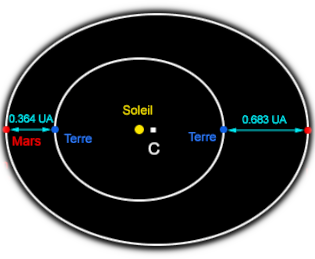Automatic translation Category: Planets and dwarf planets
Updated October 22, 2021### Image : T2 / R3 = k The theoretical expression of k will be known in 1687 thanks to the equations of universal gravitation formulated by Isaac Newton (1643−1727).### Image: Cassini measurements made it possible to measure the angle α. Two observers are placed at two sufficiently distant points on the Earth (Paris and Cayenne for example) and note the configuration of the stars surrounding the observed star. They can thus measure the angles from the observation points then deduce the parallax α (in radians) then the Earth-Mars distance.

nota: Latitude is the north or south position of a point on Earth, in other words it is the angle with the equatorial plane (from 0 ° at the equator to 90 ° at the north pole and -90 ° at the south pole).

nota: Longitude is the East-West position of a point on Earth, which corresponds to a time difference with the plane of the Greenwich meridian (prime meridian, 0 ° longitude). This shift varies from + 180 ° west to -180 ° east).1997 © Astronoo.com − Astronomy, Astrophysics, Evolution and Earth science.